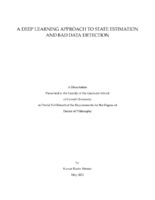## A Deep Learning Approach to State Estimation and Bad Data Detection##### Abstract
A deep neural network is a deep learning algorithm that uses artificial neural networks with multiple layers. The goal of a deep neural network is to learn a function from observation samples. In many real-world problems, this function is unknown and there is a need to learn it from the input-output samples. This idea may apply to various interesting problems that require a deep learning approach. I studied three learning problems motivated by the applications in power systems, The first problem considered is the problem of state estimation for unobservable distribution systems. A deep learning approach to Bayesian state estimation is proposed for real-time applications. The proposed technique consists of distribution learning for stochastic power injection, a Monte Carlo technique for the training of a deep neural network for state estimation, and a Bayesian bad data detection and filtering algorithm. Simulations illustrate the accuracy of Bayesian state estimation for unobservable systems and demonstrate the benefit of employing a deep neural network. Comparing with pseudo-measurement techniques, direct Bayesian state estimation via deep learning neural network outperforms existing benchmarks. The second problem considered is to detect anomalies under unknown probability distributions. Whereas the probability distribution of the anomaly-free data is unknown, anomaly-free training samples are assumed to be available. For anomaly data, neither the underlying probability distribution is known nor anomaly data samples are available. A deep learning approach coupled with a statistical test based on coincidence is proposed where an inverse generative adversary network is trained to transform data to the classical uniform vs. nonuniform hypothesis testing problem. The proposed approach is particularly effective to detect persistent anomalies whose distributions have an overlapping domain with that of the anomaly-free distribution. The third problem considered is the detection of bad-data sequences in power system. The bad-data model is nonparametric that includes arbitrary natural and adversarial data anomalies. No historical samples of data anomaly are assumed. The probability distribution of data in anomaly-free system operations is also non-parametric, unknown, but with historical training samples. A uniformity test is proposed based on a generative adversarial network (GAN) that extracts independent components of the measurement sequence via independent component analysis (ICA). Referred to as ICA-GAN, the developed approach to bad-data sequence detection can be applied at the individual sensor level or jointly at the system level. Numerical results demonstrate significant improvement over the state-of-the-art solutions for a variety of bad-data cases using PMU measurements from the EPFL smart grid testbed and that from the synthetic North Texas grid.
89 pages
2021-05
##### Keywords
Anomaly Detection; Bad Data Detection; Deep Learning; Generative Adversary Networks; ICA-GAN; State Estimation
Tong, Lang
Bitar, Eilyan
Sabuncu, Mert
##### Degree Discipline
Electrical and Computer Engineering
##### Degree Name
Ph. D., Electrical and Computer Engineering
##### Degree Level
Doctor of Philosophy
##### Types
dissertation or thesis